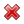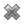# Fight Finance

#### CoursesTagsRandomAllRecentScoresScoreskeithphw $6,011.61 Jade$1,815.80 Chu $789.98 ZOE HY$763.33 royal ne... $750.00 Leehy$713.33 Visitor $650.00 JennyLI$625.61 Visitor $590.00 Visitor$555.33 Visitor $550.00 Visitor$550.00 Visitor $540.00 Visitor$500.00 Yizhou $489.18 Visitor$480.00 Visitor $480.00 Visitor$470.00 Visitor $464.70 Jasper.sun$460.00

The following cash flows are expected:

• 10 yearly payments of $80, with the first payment in 3 years from now (first payment at t=3). • 1 payment of$600 in 5 years and 6 months (t=5.5) from now.

What is the NPV of the cash flows if the discount rate is 10% given as an effective annual rate?

There are many different ways to value a firm's assets. Which of the following will NOT give the correct market value of a levered firm's assets $(V_L)$? Assume that:

• The firm is financed by listed common stock and vanilla annual fixed coupon bonds, which are both traded in a liquid market.
• The bonds' yield is equal to the coupon rate, so the bonds are issued at par. The yield curve is flat and yields are not expected to change. When bonds mature they will be rolled over by issuing the same number of new bonds with the same expected yield and coupon rate, and so on forever.
• Tax rates on the dividends and capital gains received by investors are equal, and capital gains tax is paid every year, even on unrealised gains regardless of when the asset is sold.
• There is no re-investment of the firm's cash back into the business. All of the firm's excess cash flow is paid out as dividends so real growth is zero.
• The firm operates in a mature industry with zero real growth.
• All cash flows and rates in the below equations are real (not nominal) and are expected to be stable forever. Therefore the perpetuity equation with no growth is suitable for valuation.

Where:

$$r_\text{WACC before tax} = r_D.\frac{D}{V_L} + r_{EL}.\frac{E_L}{V_L} = \text{Weighted average cost of capital before tax}$$ $$r_\text{WACC after tax} = r_D.(1-t_c).\frac{D}{V_L} + r_{EL}.\frac{E_L}{V_L} = \text{Weighted average cost of capital after tax}$$ $$NI_L=(Rev-COGS-FC-Depr-\mathbf{IntExp}).(1-t_c) = \text{Net Income Levered}$$ $$CFFA_L=NI_L+Depr-CapEx - \varDelta NWC+\mathbf{IntExp} = \text{Cash Flow From Assets Levered}$$ $$NI_U=(Rev-COGS-FC-Depr).(1-t_c) = \text{Net Income Unlevered}$$ $$CFFA_U=NI_U+Depr-CapEx - \varDelta NWC= \text{Cash Flow From Assets Unlevered}$$

Some financially minded people insist on a prenuptial agreement before committing to marry their partner. This agreement states how the couple's assets should be divided in case they divorce. Prenuptial agreements are designed to give the richer partner more of the couples' assets if they divorce, thus maximising the richer partner's:

A levered firm has zero-coupon bonds which mature in one year and have a combined face value of $9.9m. Investors are risk-neutral and therefore all debt and equity holders demand the same required return of 10% pa. In one year the firm's assets will be worth: •$13.2m with probability 0.5 in the good state of the world, or
• $6.6m with probability 0.5 in the bad state of the world. A new project presents itself which requires an investment of$2m and will provide a certain cash flow of $3.3m in one year. The firm doesn't have any excess cash to make the initial$2m investment, but the funds can be raised from shareholders through a fairly priced rights issue. Ignore all transaction costs.

Should shareholders vote to proceed with the project and equity raising? What will be the gain in shareholder wealth if they decide to proceed?

What is the present value of a nominal payment of \$1,000 in 4 years? The nominal discount rate is 8% pa and the inflation rate is 2% pa.

A stock's required total return will increase when its:

The efficient markets hypothesis (EMH) and no-arbitrage pricing theory is most closely related to which of the following concepts?

A put option written on a risky non-dividend paying stock will mature in one month. As is normal, assume that the option's exercise price is non-zero and positive $(K>0)$ and the stock has limited liability $(S>0)$.

Which of the following statements is NOT correct? The put option's:

According to the impossible trinity, a currency can only have two of these three desirable traits: be fixed against the USD; convertible to and from USD for traders and investors so there are open goods, services and capital markets; and allow independent monetary policy set by the country’s central bank, independent of the US central bank.

Which of the following exchange rate regimes sacrifices fixing the exchange rate to the USD? In other words, which regime uses a floating exchange rate?

Question 903  option, Black-Scholes-Merton option pricing, option on stock index

A six month European-style call option on the S&P500 stock index has a strike price of 2800 points.

The underlying S&P500 stock index currently trades at 2700 points, has a continuously compounded dividend yield of 2% pa and a standard deviation of continuously compounded returns of 25% pa.

The risk-free interest rate is 5% pa continuously compounded.

Use the Black-Scholes-Merton formula to calculate the option price. The call option price now is: